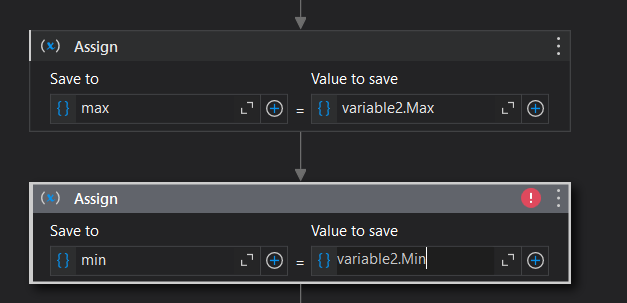# Minmax

i have one aray
stringarr={"a’,“b”,“1”,“c”,“d”,“10”,“6”,“p”,“15”}
how to find min and max number in that array
without using condition
if (int.TryParse(item, out int result))
And how to convert number in array of string without list
@Gokul001

Hi,

Max

``````arrStr.Where(Function(s) s.IsNumeric).Max(Function(s) CInt(s))
``````

or

``````arrStr.Where(Function(s) System.Text.RegularExpressions.Regex.IsMatch(s,"^\d+\$")).Max(Function(s) CInt(s))
``````

Min

``````arrStr.Where(Function(s) s.IsNumeric).Min(Function(s) CInt(s))
``````

or

``````arrStr.Where(Function(s) System.Text.RegularExpressions.Regex.IsMatch(s,"^\d+\$")).Min(Function(s) CInt(s))
``````

Regards,

string arr = { “abc”, “2”, “ghd”, “34”, “78”, “fef”, “70” };
List list = new List();
foreach (string item in arr)
{
if (int.TryParse(item, out int result))
{

``````            }
}
int[] num = list.ToArray();
int min = num;
int max = num;
foreach (var item in num)
{
if (item < min)
{
min = item;
}
if (item > max)
{
max = item;
}

}
Console.WriteLine("Minimum no is=" + min);
Console.WriteLine("Maximun num is=" + max);
``````

in that code i want to change if condition so how to change that

@purnima_mandanHi,

``````string[] arr = { "abc", "2", "ghd", "34", "78", "fef", "70" };
List<int> list = new List<int>();
foreach (string item in arr)
{
if (item.IsNumeric())
{
}
}
int[] num = list.ToArray();
int min = num;
int max = num;
foreach (var item in num)
{
if (item < min)
{
min = item;
}
if (item > max)
{
max = item;
}
}
Console.WriteLine("Minimum no is=" + min.ToString());
Console.WriteLine("Maximun num is=" + max.ToString());
``````

Sequence.xaml (5.0 KB)

Regards,

Isnumeric is not a valid expression i got error

HI,

Can you share your xaml file?

Regards,

yes but i also want answer in c# language so how to do that
and what other way to convert number

Hi,

``````if (System.Text.RegularExpressions.Regex.IsMatch(item,@"^\d+\$"))
``````

Regards,

It is done but you have any other Easy way to do that ?

The following will also work. Can you try this?

``````if (Microsoft.VisualBasic.Information.IsNumeric(item))
``````

Or do you want to achieve it without “if”?

Regards,

How to do withput if condition

For example, use filter as the following.

``````string[] arr = { "abc", "2", "ghd", "34", "78", "fef", "70" };
int[] num = arr.Where(s=> System.Text.RegularExpressions.Regex.IsMatch(s,@"^\d+\$")).Select(s=> Int32.Parse(s)).ToArray();
``````

how to do this programme using char.isdigit function in if condition
and what is different way to define string of array

``````if (item.All(c=>Char.IsDigit(c)))
``````

and what is different way to define string of array